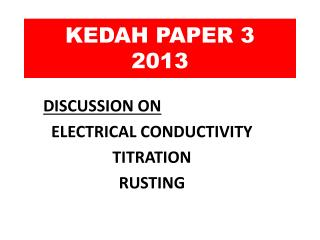DownloadDownload PresentationKEDAH PAPER 3 2013

# KEDAH PAPER 3 2013

Télécharger la présentation## KEDAH PAPER 3 2013

- - - - - - - - - - - - - - - - - - - - - - - - - - - E N D - - - - - - - - - - - - - - - - - - - - - - - - - - -
##### Presentation Transcript

1. KEDAH PAPER 32013 DISCUSSION ON ELECTRICAL CONDUCTIVITY TITRATION RUSTING

2. A student carried out three experiments to investigate the electrical conductivity of three compounds, that is naphthalene, lead(II)bromide and glucose in their molten state. Diagram 1.1 shows the results obtained from the experiment.

3. Carbon Carbon Molten naphthalene

4. b) Based on the observations in Diagram 1.1, state the inference for these experiments. Molten lead(II) bromide can conduct electricity while molten naphthalene and glucose cannot conduct electricity. notes inference is conclusion from observation. Do not give theory!

5. a)Construct a table to record results for this exp Note: If reading has 5 sections, then read to one decimal place, If 10 sections , then read to two dec places

6. c) State operational definition for the electrical conductivity. • Note: operational def must have • is/ has/ forms/shows • what you observe, • ii) from what procedure • Electrical conductivity shows ammeter needle deflected when the molten lead(II) bromide is electrolysed using carbon electrodes

7. notes c) State one hypothesis for this experiment. Note: hypothesis must contain i) early conclusion from aim of the exp and ii) must relate to MV and RV Aim : to investigate the electrical conductivity of three compounds, that is naphthalene, lead(II)bromide and glucose in their molten state. MV : naphthalene, lead(II)bromide and glucose RV : electrical conductivity

8. c) State one hypothesis for this exp. Aim : to investigate the electrical conductivity of three compounds, that is naphthalene, lead(II)bromide and glucose in their molten state. ANSWER lead(II)bromide in molten state can conduct electricity while naphthalene and glucose cannot conduct electricity MV : naphthalene, lead(II)bromide and glucose RV: electrical conductivity

9. d) State all the variables for this exp. Manipulated variable: naphthalene, lead(II)bromide and glucose Responding variable Ammeter reading OR Deflection of needle . Constant variable Molten state OR Carbon electrodes

10. Compounds can be classified into ionic compound and covalent compound.Based on the compounds in Diagram 1.1, complete Table 1 by classifying the compounds into ionic or covalent compounds. notes Note: i) Ionic compound= metal atom + non metal atom ii) covalent compound = non metal atom + non metal atom

11. 2 Diagram 2.1 shows a titration method used to determine the concentration of potassium hydroxide solution.

12. Acid base indicator notes

13. a)Based on Diagram 2.1, sulphuric acid is added gradually to potassium hydroxide solution while swirling until the mixture in the conical flask change colour. State the colour change Alkali to neutral ANSWER:Pink to colourless

14. (b) Name the type of reaction between potassium hydroxide solution and sulphuric acid. • Types of reaction • Acid + alkali Answer: Neutralisation

15. notes Types of reaction neutralisation Precipitation reaction / double decomposition Displacement of metal Displacement of halogen Combustion Decomposition

16. notes State type of reactions a) Silver nitrate + sodium chloride Precipitation reaction b) Nitric acid + potasium hydroxide Neutralisation c) Chlorine + sodium iodide Displacement of halogen d) Zinc + copper(II) sulphate Displacement of metal e) Butanol + excess oxygen Combustion f) copper(II) carbonate  copper(II) oxide + CO2 Decomposition g) Hydrogen peroxide  oxygen + water decomposition

17. (c) Table 2.2 shows the volume of sulphuric acid used in the titration Calculate the (i) average volume of sulphuric acid used. Average volume of sulphuric acid = 10.30 + 10.40 + 10.20 3 = 10.30 cm3

18. notes (ii) Calculate concentration of potassium hydroxide solution • Follow steps • Write balanced equation • Calculate mol • Ratio • Change to required unit

19. 2KOH (aq) + H2SO4 (aq) K2SO4 (aq) + 2H2O (l) Mol of sulphuric acid = MV/1000 = 1x 10.3/1000 = 0.0103 mol (ii) concentration of potassium hydroxide solution From equation, 1 mol of H2SO4 reacts with 2 moles KOH So, 0.0103 mol H2SO4 0.0206 moles KOH Mole of KOH = MV/1000 So M= mole x 1000/V = 0.0206 x 1000/25 =0.824 mol dm-3

20. notes You can check using formula for neutralization (ii) concentration of potassium hydroxide solution Ma x [H+] x Va = Mb x [OH-] x Vb So Mb = Ma x [H+] x Va Vb x [ OH -] Concentration of KOH = 1.0 x 2 x 10.30 25.0 x 1 = 0.82 mol dm-3

21. notes (d)The experiment is repeated with 1.0 moldm -3 nitric acid.Predict the average volume of nitric acid needed for the titration with potassium hydroxide solution. • Predict can either be • In the form of value, ( if value is used) • In the form of ‘ more than, less than or no change’ (if value cannot be used)

22. notes (d)The experiment is repeated with 1.0 moldm -3 nitric acid.Predict the average vol of nitric acid needed for the titration with potassium hydroxide solution. Sulphuric acid is a diprotic acid , contains 2 mol of hydrogen ion per 1 mol of acid Nitric acid is a monoprotic acid contains only 1 mol of hydrogen ion per 1 mol of acid So if HNO3 is used to replace H2SO4, then the volume is double Use value! Answer 20.60 cm3